keras 训练大数据_keras model训练 添加数据后接着训练 - CSDN
• 转处：http://blog.csdn.net/lujiandong1/article/details/54869170说明：我是在keras的官方demo上进行修改https://github.com/fchollet/keras/blob/master/examples/imdb_cnn.py1、几点说明，从文件中读入数据，会...
转处：http://blog.csdn.net/lujiandong1/article/details/54869170说明：我是在keras的官方demo上进行修改https://github.com/fchollet/keras/blob/master/examples/imdb_cnn.py1、几点说明，从文件中读入数据，会降低GPU的使用率，如果能够直接将数据载入内存，GPU的使用率会比较高。下面进行对比：全部数据载入内存,GPU的使用率：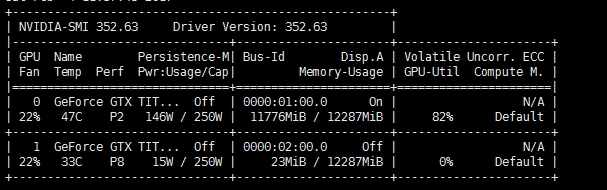使用队列,边读数据边进行训练：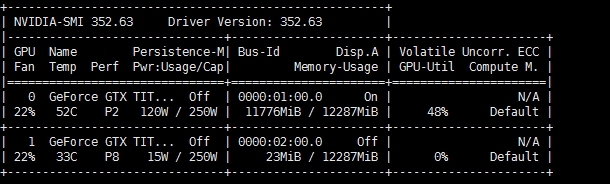结论：全部载入内存，GPU的使用率可以达到82%,如果边载入数据边训练,只能达到48%2、keras 使用迭代器来实现大数据的训练,其简单的思想就是,使用迭代器从文件中去顺序读取数据。所以,自己的训练数据一定要先随机打散。因为，我们的迭代器也是每次顺序读取一个batch_size的数据进行训练。举例如下：数据如下,前400维是特征,后一维是label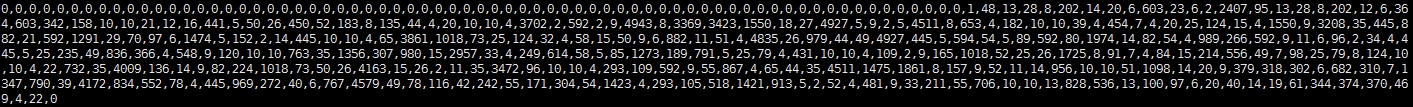keras 官方的demo 如下:[python] view plain copydef generate_arrays_from_file(path):      while 1:      f = open(path)      for line in f:          # create Numpy arrays of input data          # and labels, from each line in the file          x, y = process_line(line)          yield (x, y)      f.close()    model.fit_generator(generate_arrays_from_file('/my_file.txt'),          samples_per_epoch=10000, nb_epoch=10)  说明：官方的demo还是有瑕疵的,没有实现batch_size,该demo每次只能提取一个样本。我针对上述的数据集,实现的batch_size数据提取的迭代器,代码如下：[python] view plain copydef process_line(line):      tmp = [int(val) for val in line.strip().split(',')]      x = np.array(tmp[:-1])      y = np.array(tmp[-1:])      return x,y    def generate_arrays_from_file(path,batch_size):      while 1:          f = open(path)          cnt = 0          X =[]          Y =[]          for line in f:              # create Numpy arrays of input data              # and labels, from each line in the file              x, y = process_line(line)              X.append(x)              Y.append(y)              cnt += 1              if cnt==batch_size:                  cnt = 0                  yield (np.array(X), np.array(Y))                  X = []                  Y = []      f.close()  训练时候的代码如下：[python] view plain copymodel.fit_generator(generate_arrays_from_file('./train',batch_size=batch_size),          samples_per_epoch=25024,nb_epoch=nb_epoch,validation_data=(X_test, y_test),max_q_size=1000,verbose=1,nb_worker=1)  3、关于samples_per_epoch的说明：我的训练数据,train只有25000行,batch_size=32。照理说samples_per_epoch=32,但是会有警告.UserWarning: Epoch comprised more than samples_per_epoch samples, which might affect learning results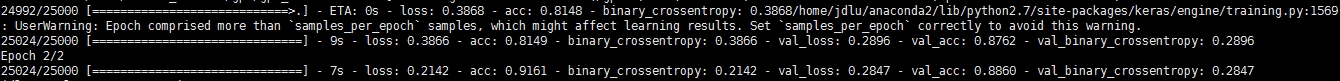说明：这个出错的原因是train的数目/batch_size不是整数。可以将samples_per_epoch = ceil(train_num/batch_size) *batch_size.设置完的结果为88.72%：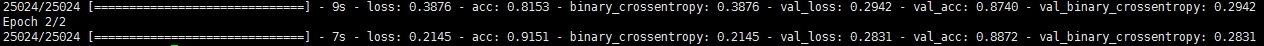keras的demo使用的方法是将全部数据载入进来训练: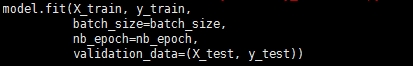demo的结果为88.86%，所以，该数据读取的方式基本没问题。但是，一定要将数据先进行打乱。如果能全部载入内存，就全部载入内存，速度会快不少
展开全文• 官方提供的.flow_from_directory(directory)函数可以读取并训练大规模训练数据，基本可以满足大部分需求，可以参考我的笔记。但是在有些场合下，需要自己读取大规模数据以及对应标签，下面提供一种方法。这个方法是...
简介

官方提供的.flow_from_directory(directory)函数可以读取并训练大规模训练数据，基本可以满足大部分需求，可以参考我的笔记。但是在有些场合下，需要自己读取大规模数据以及对应标签，下面提供一种方法。这个方法是读取图片的地址保存到内存中，这样就不会出现内存不足情况了。

下面我使用的数据集是猫狗的数据集，下载地址

猫狗数据集：https://pan.baidu.com/s/13hw4LK8ihR6-6-8mpjLKDA   密码：dmp4

代码

from keras.preprocessing.image import load_img, img_to_array
from keras.layers import Conv2D, MaxPooling2D, Dropout, Dense
from keras.layers.core import Activation, Flatten
from keras.models import Sequential
from keras.optimizers import RMSprop

import numpy as np
import os
import random

train_file_path = 'kaggle/train/'

val_x = []
train_x = []
count = 0
for dir, file, images in os.walk(train_file_path):
for image in images:
# print(image)
count += 1
fullname = os.path.join(dir, image)
if count%5 == 0:
val_x.append(fullname)
train_x.append(fullname)

# print(len(train_x))
#
# for i, val in enumerate(train_x):
#     print(val)
#     if i == 10:
#         break

def get_image_label(image_paths):
# print(image_paths)
image_labels = []
for image_path in image_paths:
# print(image_path)
image_name = image_path.split('/')
# print(image_name)
if 'cat' in image_name:
image_labels.append(0)
else:
image_labels.append(1)
# break
return image_labels

# image_labels = get_image_label(train_x)
# for i, image_label in enumerate(image_labels):
#     print(image_label)
#     if i == 10:
#         break

# 读取图片
def load_batch_image(img_path, train_set = True, target_size=(150, 150)):
if train_set:
return img_to_array(im)  # converts image to numpy array
else:
return img_to_array(im) / 255.0

# 建立一个数据迭代器
def GET_DATASET_SHUFFLE(X_samples, batch_size, train_set = True):
random.shuffle(X_samples)
# for i, image_label in enumerate(X_samples):
#     print(image_label)
#     if i == 10:
#         break

batch_num = int(len(X_samples) / batch_size)
max_len = batch_num * batch_size
X_samples = np.array(X_samples[:max_len])
y_samples = np.array(get_image_label(X_samples))

print('X_samples.shape:', X_samples.shape)

X_batches = np.split(X_samples, batch_num)
# print(X_batches)
# for x_batch in X_batches:
#     print(x_batch)
#     break
y_batches = np.split(y_samples, batch_num)

# for i, y_batch in y_batches:
#     print('y_batch:', y_batch)
#     if i == 10:
#         break
# print('y_batches:', y_batches)

for i in range(len(X_batches)):
if train_set:
x = np.array(list(map(load_batch_image, X_batches[i], [True for _ in range(batch_size)])))
else:
x = np.array(list(map(load_batch_image, X_batches[i], [False for _ in range(batch_size)])))
# print(x.shape)
y = np.array(y_batches[i])
yield x, y

# 搭建模型
model = Sequential()
input_shape=(150, 150, 3)))

print(model.summary())

model.compile(loss='binary_crossentropy',
optimizer=RMSprop(lr=1e-4),
metrics=['acc'])

batch_size = 32

model.fit_generator(
GET_DATASET_SHUFFLE(train_x, batch_size, True),
epochs=10,
steps_per_epoch=int(len(train_x)/batch_size))

里面有很多被注释的测试程序， 你可以做一下参考吧

参考博客地址：http://www.cnblogs.com/hejunlin1992/p/9371078.html


展开全文• 在本文中，我们将讨论如何使用Keras在不适合内存的大数据集上训练我们的深度学习网络。 介绍 深度学习算法优于所有其他算法，能够在大多数问题上产生最先进的结果。深度学习算法成功的主要原因是数据集的大小...
https://www.toutiao.com/a6670173759829180936/在本文中，我们将讨论如何使用Keras在不适合内存的大数据集上训练我们的深度学习网络。

介绍

深度学习算法优于所有其他算法，能够在大多数问题上产生最先进的结果。深度学习算法成功的主要原因是数据集的大小越来越大。现在，深度学习算法在大型数据集上进行训练，这些数据集甚至不适合内存。问题是：如何在如此庞大的数据集上训练我们的模型？本文分为以下几个部分：

一般情况下，深度学习算法的性能优于其他算法，并且能够在大多数问题上产生较好的结果。深度学习算法成功的主要原因是数据集的不断增大。现在，深度学习算法在大型数据集上进行训练，这些数据集甚至不适合内存。问题是:如何在如此巨的数据集中训练我们的深度学习模型呢?本文分为以下几个部分:

下载和了解数据集
数据集的准备 - 批量加载数据集
数据集在训练和验证集中的Shuffling和拆分
创建自定义生成器
定义模型体系结构和训练模型
结论
作为一个例子，我们将解决Kaggle“Plant Seedlings Classification”的挑战。这个数据集并不大，但我们将假设数据集太大，无法装入内存，然后将批量加载数据集。

下载和了解数据集

您可以从此处下载(https://www.kaggle.com/c/plant-seedlings-classification/data)数据集。解压缩train.zip文件夹。该数据集包含分类为12种植物物种的不同阶段的植物幼苗的4750幅图像。12种植物有Black-grass，Charlock，Cleavers，Common Chickweed，Common wheat，Fat Hen，Losse Silky-bent，Maize，Scentless Mayweed，Shepherds Purse，Small-flowered Cranesbill，Sugar beet。竞赛的目标是创建一个能够从照片中确定植物种类的分类器。

当前目录如下所示：文件夹目录

如果您的问题数据集不是这种格式，请不要担心。您的数据集可以是任何格式。我们将在下一节中看到，目标是获取所有数据点（即我们示例中的图像）并将它们保存到单个文件夹中。数据点可以是图像，音频等。

数据集的准备 - 批量加载数据集

下一步是获取整个数据集（即所有数据点（在我们的示例中为图像））并将它们存储到一个文件夹中。我们创建一个名为“all_images”的新文件夹，目标是将数据集中的所有图像存储在这个“all_images”文件夹中。

我们使用以下脚本将所有图像存储在“all_images”文件夹中。您可以编写类似的脚本来获取数据集中的所有数据点（可以是图像，音频等）并将它们存储到新文件夹中。

import os
import shutil
train_dir = '/content/train'
dest_dir = '/content/all_images'
counter = 0
for subdir, dirs, files in os.walk(train_dir):
#print(files)
for file in files:
full_path = os.path.join(subdir, file)
shutil.copy(full_path, dest_dir)
counter = counter + 1
print(counter)下一步是将每个数据点的名称(即每个图像的名称)存储在一个数组中(让我们将数组命名为filename)。还有一件事是将与每个数据点关联的标签存储在另一个数组中(让我们将这个数组称为labels)。

下面是一个Python脚本，它将每个图像的名称存储在filenames数组中，并将与该图像关联的标签存储在labels数组中。

注意：请记住，每个数据点的名称应该是唯一的。

import numpy as np
subdirs, dirs, files = os.walk('/content/all_images').__next__()
m = len(files)
print(m)
filenames = []
labels = np.zeros((m, 1))
import os
import shutil
images_dir = '/content/all_images'
filenames_counter = 0
labels_counter = -1
for subdir, dirs, files in os.walk(train_dir):
#print(files)
for file in files:
filenames.append(file)
labels[filenames_counter, 0] = labels_counter
filenames_counter = filenames_counter + 1
labels_counter = labels_counter+1

print(len(filenames))
print(labels.shape)现在，您可以保存“all_images”文件夹、“filename”数组和“labels”数组，以便稍后使用。

下面我们创建一个由filename 和labels组成的numpy数组，并将它们保存为.npy文件。

# saving the filename array as .npy file
np.save('filenames.npy', filenames)
import keras
from keras.utils import to_categorical
# One hot vector representation of labels
y_labels_one_hot = to_categorical(labels)
# saving the y_labels_one_hot array as a .npy file
np.save('y_labels_one_hot.npy', y_labels_one_hot)数据集的shuffling和拆分

下一步是对数据集进行shuffle ，以便从数据集中删除对称性。

from sklearn.utils import shuffle
filenames_shuffled, y_labels_one_hot_shuffled = shuffle(filenames, y_labels_one_hot)
# saving the shuffled file.
np.save('y_labels_one_hot_shuffled.npy', y_labels_one_hot_shuffled)
np.save('filenames_shuffled.npy', filenames_shuffled)现在，让我们将数据集拆分为训练和验证集。我们也可以保存这些文件，因为这些文件稍后将用于训练和验证我们的机器学习模型。

from sklearn.model_selection import train_test_split
filenames_shuffled_numpy = np.array(filenames_shuffled)
X_train_filenames, X_val_filenames, y_train, y_val = train_test_split(
filenames_shuffled_numpy, y_labels_one_hot_shuffled, test_size=0.2, random_state=1)
print(X_train_filenames.shape)
print(y_train.shape)
print(X_val_filenames.shape)
print(y_val.shape)
np.save('X_train_filenames.npy', X_train_filenames)
np.save('y_train.npy', y_train)
np.save('X_val_filenames.npy', X_val_filenames)
np.save('y_val.npy', y_val)您还可以将“all_images”文件夹保存为zip格式，以便与其他团队成员共享数据集。

这些代码行只创建一个“all_images.zip”文件夹。

import shutil
shutil.make_archive("all_images", "zip", "all_images")创建自定义生成器

注意：由于我们的数据集太大而无法容纳在内存中，因此我们必须将数据集从硬盘批量加载到内存中。

为此，我们将创建一个自定义生成器。我们的自定义生成器将要将数据集从硬盘批量加载到内存中。

class My_Custom_Generator(keras.utils.Sequence) :

def __init__(self, image_filenames, labels, batch_size) :
self.image_filenames = image_filenames
self.labels = labels
self.batch_size = batch_size

def __len__(self) :
return (np.ceil(len(self.image_filenames) / float(self.batch_size))).astype(np.int)

def __getitem__(self, idx) :
batch_x = self.image_filenames[idx * self.batch_size : (idx+1) * self.batch_size]
batch_y = self.labels[idx * self.batch_size : (idx+1) * self.batch_size]

return np.array([
resize(imread('/content/all_images/' + str(file_name)), (80, 80, 3))
for file_name in batch_x])/255.0, np.array(batch_y)让我们试着理解整个代码：

第1行：我们的Custom Generator类继承自Sequence类。
第3行：在这里，我们可以向生成器提供参数。
第9行：此函数计算此生成器应该生成的批数。因此，我们将总样本数除以batch_size并返回该值。
第14行：在这里，给定批号idx，您需要将包含数据批次和ground-truth（GT）的列表放在一起。在此示例中，我们读取大小为batch_size的批量图像，并返回一个数组[image_batch, GT]。
在__getitem __（self，idx）函数中，您可以决定批量加载时数据集会发生什么。在这里，您也可以执行预处理步骤。此外，您还可以计算音频文件的mel谱图。
我们已经创建了数据生成器。下一步是创建此类的实例。

batch_size = 32
my_training_batch_generator = My_Custom_Generator(X_train_filenames, y_train, batch_size)
my_validation_batch_generator = My_Custom_Generator(X_val_filenames, y_val, batch_size)第3,4行：实例化My_Custom_Generator的两个实例（一个用于训练，一个用于验证）。
定义模型体系结构和训练模型

我们先导入一些Python库：

import numpy as np
import pandas as pd
from skimage.transform import resize
import keras
from keras.models import Sequential, Model, load_model
from keras.layers import Input, Conv1D, Conv2D, MaxPooling1D, MaxPooling2D, Dense, Dropout, Activation, Flatten
from keras.layers.normalization import BatchNormalization
from keras.utils import to_categorical现在，让我们定义模型架构并编译机器学习模型。您可以在此处使用任何模型架构。

model = Sequential()
model.add(Conv2D(filters = 64, kernel_size = (5,5), activation ='relu',input_shape=(80,80,3)))
model.add(Conv2D(filters = 64, kernel_size = (5,5), activation ='relu'))
model.add(Conv2D(filters = 128, kernel_size = (5,5), activation ='relu'))
model.add(Conv2D(filters = 128, kernel_size = (5,5), activation ='relu'))
model.add(Conv2D(filters = 256, kernel_size = (5,5), activation ='relu'))
model.add(Conv2D(filters = 256, kernel_size = (5,5), activation ='relu'))
model.add(Dense(256, activation = "relu")) #Fully connected layer
model.add(Dense(60, activation = "relu")) #Fully connected layer
model.add(Dense(12, activation = "softmax")) #Classification layer or output layer
model.summary()现在，让我们训练我们的机器学习模型。

model.fit_generator(generator=my_training_batch_generator,
steps_per_epoch = int(3800 // batch_size),
epochs = 10,
verbose = 1,
validation_data = my_validation_batch_generator,
validation_steps = int(950 // batch_size))训练结果：我们的模型没有给出很好的结果，而且过度拟合。我们可以使用不同的模型架构，或者预处理我们的数据集，或使用数据增强，或者使用迁移学习来提高精度。但是，这不是本文的目的。我希望现在您已经非常清楚地理解了如何使用大数据集来训练一个深度学习模型。

结论

这篇文章，我试着用一个例子给你一个非常清晰的理解，告诉你如何用一个巨大的数据集训练你自己的深度学习模型。

注意：最好先对数据集进行预处理，然后将其提供给学习算法。

展开全文Keras
• 参考1 参考2 train_on_batch
参考1
参考2
train_on_batch

n_epoch = 12
batch_size = 16
for e in range(n_epoch):
print("epoch", e)
batch_num = 0
loss_sum=np.array([0.0,0.0])
for X_train, y_train in GET_DATASET_SHUFFLE(train_X, batch_size, True): # chunks of 100 images
for X_batch, y_batch in train_datagen.flow(X_train, y_train, batch_size=batch_size): # chunks of 32 samples
loss = model.train_on_batch(X_batch, y_batch)
loss_sum += loss
batch_num += 1
break #手动break
if batch_num%200==0:
print("epoch %s, batch %s: train_loss = %.4f, train_acc = %.4f"%(e, batch_num, loss_sum/200, loss_sum/200))
loss_sum=np.array([0.0,0.0])
res = model.evaluate_generator(GET_DATASET_SHUFFLE(val_X, batch_size, False),int(len(val_X)/batch_size))
print("val_loss = %.4f, val_acc = %.4f: "%( res, res))

model.save("weight.h5")



展开全文• 以前做的练手小项目导致新手产生一个惯性思维——读取训练集图片的时候把所有图读到内存中，然后分批训练。 其实这是有问题的，很容易导致OOM。现在内存一般16G，而训练集图片通常是上万张，而且RGB图，还很大，VGG...
由于paper中的数据量过大，从自己的mac换到导师的办公室电脑，再换到学院的服务器（最后被我整挂了，谢谢老师没有怪我），还是提示memory error，之后上网搜了关于大数据内存溢出的问题，只有一篇教程仅贴出代码，所以我打算记录下来我学习的过程。
（！！！提示：由于我不是计算机专业的学生，代码也都是自己学的，有出错的地方请给我留言，我马上改正）
首先，在读入图片数据时，之前由于数据量小，都是直接全部读入，也没有改代码，所以导致碰到大数据量的时候，总是直接down掉。因此，我们需要分批导入，并且同时后续的需要分批训练。
情况一：train set及validation set已分

"""
Created on Fri Sep  7 10:25:27 2018

@author: Cathy.S
"""

def get_batch(X_train, y_train, img_w, img_h, color_type, batch_size, capacity):
'''
Args:
X_train: train img path list #图片训练集路径的list
y_train: train labels list #图片训练集的标签的list
img_w: image width #图片的宽
img_h: image height #图片的高
batch_size: batch size #批量大小
capacity: the maximum elements in queue #队伍中元素的最大值
Returns:
X_train_batch: 4D tensor [batch_size, width, height, chanel],\
dtype=tf.float32 #返回的是4维tensor，即[一批中包含的图片数量，图片宽，图片高，信道（一般为3）]
y_train_batch: 1D tensor [batch_size], dtype=int32 #返回的是1维tensor，即一批中包含的图片数量
'''

X_train = tf.cast(X_train, tf.string) #转换类型：转换为string

y_train = tf.cast(y_train, tf.int32) #转换类型：转换为int32

# make an input queue
'''
tf.train.slice_input_producer是一个tensor生成器
作用是按照设定，每次从一个tensor列表中按顺序或者随机抽取出一个tensor放入文件名队列
第一位参数包含一系列tensor的列表，表中tensor的第一维度的值必须相等，即个数必须相等，有多少个图像，就应该有多少个对应的标签。
'''
input_queue = tf.train.slice_input_producer([X_train, y_train])

y_train = input_queue
X_train = tf.image.decode_jpeg(X_train_contents, channels=color_type)

X_train = tf.image.resize_images(X_train, [img_h, img_w],
tf.image.ResizeMethod.NEAREST_NEIGHBOR)

X_train_batch, y_train_batch = tf.train.batch([X_train, y_train],
batch_size=batch_size,
capacity=capacity)
y_train_batch = tf.one_hot(y_train_batch, 10) #这里可能会出错

return X_train_batch, y_train_batch


跑的时候的代码如下：

import os
import tensorflow as tf
import pandas as pd
#得到X_train
path1 = "E:/paper3-XXX/20180907/pic/train" #路径
X_train = [os.path.join(path1, f) for f in os.listdir(path1)]  #路径中的文件（图片）
#得到y_train
#这里我们要消除一个x与y对应的问题（例如：X_train中是pic1，pic10，pic11这样排序，所以y_train中也要按照这个重新排序）
imds_y=[]
for img in X_train:
imds_y.append(img) #是存储图片文件名的list
y_train['Site'] = y_train['Site'].astype('category')
y_train['Site'].cat.reorder_categories(imds_y, inplace=True)
y_train.sort_values('Site', inplace=True)
y_train = y_train.drop(['site'],axis=1) #由于我的标签的表格中第一列是Site，所以去除掉
#得到color_type，img_h，img_w，即图片的尺寸，我的是224*224*3
color_type = 3
img_h = 224
img_w = 224
#得到batch_size, capacity
train_batch_size = ???
capacity = ???
#这里可以自己设。

X_train_batch=[]
y_train_batch=[]
X_train_batch, y_train_batch = get_batch(X_train, y_train,
img_w, img_h, color_type,
train_batch_size, capacity)

#同理可得validation set
#得到X_valid
path2 = "E:/paper3-XXX/20180907/pic/valid" #路径
X_valid = [os.path.join(path2, f) for f in os.listdir(path2)]  #路径中的文件（图片）
#得到y_valid
imds_y=[]
for img in X_valid:
imds_y.append(img) #是存储图片文件名的list
y_valid['Site'] = y_valid['Site'].astype('category')
y_valid['Site'].cat.reorder_categories(imds_y, inplace=True)
y_valid.sort_values('Site', inplace=True)
y_valid = y_valid.drop(['site'],axis=1) #由于我的标签的表格中第一列是Site，所以去除掉
#得到batch_size
valid_batch_size = ???

X_valid_batch=[]
y_valid_batch=[]
X_valid_batch, y_valid_batch = get_batch(X_valid, y_valid,
img_w, img_h, color_type,
valid_batch_size, capacity)

情况二：train set及validation set未分
其实和情况一差不多，先划分再分批和先分批再划分的效果是一样的，只是加了一步而已。
同样先定义get_batch，同上代码
之后如下：

import os
import tensorflow as tf
import pandas as pd
#得到X_data
path3 = "E:/paper3-XXX/20180907/pic" #路径
X_data = [os.path.join(path3, f) for f in os.listdir(path3)]  #路径中的文件（图片）
#得到y_data
imds_y=[]
for img in X_data:
imds_y.append(img) #是存储图片文件名的list
y_data['Site'] = y_data['Site'].astype('category')
y_data['Site'].cat.reorder_categories(imds_y, inplace=True)
y_data.sort_values('Site', inplace=True)
y_data = y_data.drop(['site'],axis=1) #由于我的标签的表格中第一列是Site，所以去除掉
#得到color_type，img_h，img_w，即图片的尺寸，我的是224*224*3
color_type = 3
img_h = 224
img_w = 224
#得到batch_size, capacity
data_batch_size = ???
capacity = ???
#这里可以自己设。

#分批读取
X_data_batch=[]
y_data_batch=[]
X_data_batch, y_data_batch = get_batch(X_data, y_data,
img_w, img_h, color_type,
data_batch_size, capacity)

#划分train set和validation set
"""
这里不能用train_test_split，因为现有的data是tensor，如果像平常一样是array或list就可以
"""
tf.split_v(tf.random_shuffle(



还未改完其他，待续！
展开全文tensorflow keras python
• 在使用keras的model.fit(x, y)训练模型时，需要全部加载训练的数据，如果数据较小时（如mnist数据集），加载全部的数据没什么问题，但是当自己的数据集很大时（如10G），全部加载数据可能会导致内存溢出，或者仅仅...
• 哈喽。...比如自己写个next，边读边训练】，那么keras同样也可以，比如DataGenerater和fit_generator，下面我就试试我占用内存30多个G的数据。 1-首先我先把模型修改好，能训练数据。 采用tf.keras...keras
• 官方提供的.flow_from_directory(directory)函数可以读取并训练大规模训练数据，基本可以满足大部分需求。但是在有些场合下，需要自己读取大规模数据以及对应标签，下面提供一种方法。 步骤0：导入相关 import ...
• 使用Keras如果要使用大规模数据集对网络进行训练，就没办法先加载进内存再从内存直接传到显存了，除了使用Sequence类以外，还可以使用迭代器去生成数据，但迭代器无法在fit_generation里开启多进程，会影响数据的...Keras 深度学习
• 点击上方“AI公园”，关注公众号，选择加“星标“或“置顶”作者：Soumendra P编译：ronghuaiyang导读我们在学习的时候，一般会使用minist，cifa...
• keras进行大数据训练，为了加快训练，需要提前制作训练集。 由于HDF5的特性，所有数据需要一次性读入到内存中，才能保存。 为此，我采用分批次分为2个以上HDF5进行存储。 1、先读取每个标签下的图片，并设置标签 ...
• keras数据集主要有以下7种（可从keras官方文档阅读：https://keras.io/datasets/），对其中部分数据集我进行了学习和实践，并写了笔记。 另外加几个数据集的下载地址： dog images:...keras
• 说明： 1.主要参考Francois Chollet《Deep...3.数据集和预训练模型VGG-16需要手动添加； 4.卷积神经网络请参考：【深度学习】：卷积神经网络(CNN) # This Python 3 environment comes with many helpful analytics...freeze VGG 预训练模型 数据增强 微调
• 向AI转型的程序员都关注了这个号????????????机器学习AI算法工程 公众号：datayx一、运行环境的安装：1、下载好cuda9跟cudnn7，然后在安装好后，cuda其会...
• 以前做的练手小项目导致新手产生一个惯性思维——读取训练集图片的时候把所有图读到内存中，然后分批训练。其实这是有问题的，很容易导致OOM。现在内存一般16G，而训练集图片通常是上万张，而且RGB图，还很大，VGG16...
• 说明：我是在keras的官方demo上进行修改https://github.com/fchollet/keras/blob/master/examples/imdb_cnn.py 1、几点说明，从文件中读入数据，会降低GPU的使用率，如果能够直接将数据载入内存，...keras
• Keras是一个高度封装的库，它的优点是可以进行快速的建模，缺点是它不处理底层运算，如张量内积等。为了弥补这个问题，Keras提供“后端引擎”来实现底层运算操作。目前Keras支持的后端引擎有tensorflow，CNTK，...
• 在工程中，模型常常需要训练大数据，而大数据的读取通常不能一次性读取进内存中，因此需要不断从数据集中读取数据并进行处理。在大数据中，这部分的耗时相当可观，因此可以利用tfrecord进行预先处理数据，节省读取和...
...